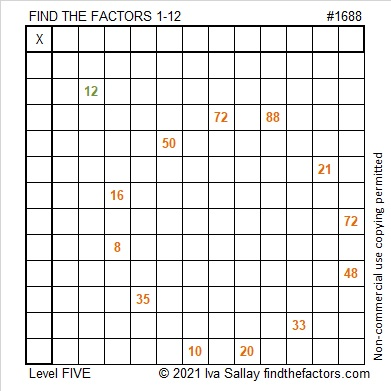# 1688 What Can Grow from a Little Seed?

Contents

### Today’s Puzzle:

Pumpkins are often harvested this time of year. It is amazing how one little seed properly planted and tended can grow into a vine that produces pumpkin after pumpkin after pumpkin.  Indeed great things can come from little things.

This level 5 puzzle starts out fairly easy. It doesn’t get very complicated until you’re about halfway through. Don’t give up! Your mind will grow as you use logic to find a way to work it out. Write the numbers from 1 to 12 in both the first column and the top row so that those numbers and the given clues make a multiplication table.### Factors of 1688:

• 1688 is a composite number.
• Prime factorization: 1688 = 2 × 2 × 2 × 211, which can be written 1688 = 2³ × 211.
• 1688 has at least one exponent greater than 1 in its prime factorization so √1688 can be simplified. Taking the factor pair from the factor pair table below with the largest square number factor, we get √1688 = (√4)(√422) = 2√422.
• The exponents in the prime factorization are 3 and 1. Adding one to each exponent and multiplying we get (3 + 1)(1 + 1) = 4 × 2 = 8. Therefore 1688 has exactly 8 factors.
• The factors of 1688 are outlined with their factor pairs in the graphic below.### More About the Number 1688:

1688 is the difference of two squares in two different ways:
423² – 421² = 1688, and
213² – 209² = 1688.

1688₁₀ = 888₁₄ because 8(14² + 14¹ + 14º) = 1688.

This site uses Akismet to reduce spam. Learn how your comment data is processed.